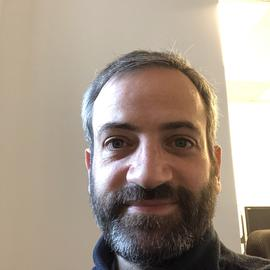# R can write R code, too

Here’s the blog post originally posted on babelgraph.org on April 14, 2012.

In a recent blog post by CMastication, a little meme puzzle is presented with the introduction that a preschooler could solve it in 5-10 minutes, a programmer in an hour. I took the bait.

The original problem goes like this:

8809=6
7111=0
2172=0
6666=4
1111=0
3213=0
7662=2
9313=1
0000=4
2222=0
3333=0
5555=0
8193=3
8096=5
7777=0
9999=4
7756=1
6855=3
9881=5
5531=0
2581=?


N.B. It turns out my strategy is completey wrong, but read on for an experiment with using eval and parse to generate code on the fly. An explanation and computational solution are available at the original site mentioned above.

## Strategy

That being said, my first guess was to look for some set of operators +,-,*,/ between each of the digits that would produce the correct response. For example, 9313=1 could be calculated as 9 / 3 + 1 – 3 = 1, giving a solution of /+-. In order to try every combination of the four basic arithmetic operators in three different positions, I had to generate some R code on the fly.

## R writes R

One of the things I like about R is that not only can I write R code, but R can write R code, too. We can create a line of code and store it as a variable. Then R will evaluate it whenever we like.

{% gist gweissman/2377829 %}

I can create the code dynamically by making a string representation of the code, then parsing it into an expression that can be evaluated as above.

{% gist /gweissman/2385949 %}

Clearly it doesn’t give us the right answer.

But it was fun to code in R with R.###### Assistant Professor of Medicine

I am a pulmonary and critical care physician at the University of Pennsylvania Perelman School of Medicine, and core faculty member at the Palliative and Advanced Illness Research (PAIR) Center. My work seeks to translate innovations in artificial intelligence methods into bedside clinical decision support systems that alleviate uncertainty in critical clinical decisions. My research interests include clinical informatics, natural language processing, machine learning, population health, and pragmatic trials.